## Section 5.1  PHYSICAL CONSTANTSTable 5-1 was drawn from the recommendations of CODATA (the Committee on Data for Science and Technology). The full 1998 CODATA set of constants may be found at http://physics.nist.gov/cuu/Constants/index.html. A PDF version of this table is also available.

 Table 5-1. Physical constants. Quantity Symbol, equation Value Uncert. (ppb) speed of light c (see note *) 2.997 924 58´108 m s–1 (1010 cm s–1) exact Planck constant h 6.626 068 76(52)´10–34 J s (10–27 erg s) 78 Planck constant, reduced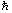= h/2p 1.054 571 596(82)´10–34 J s = 6.582 118 89(26)´10–22 MeV s 78, 39 electron charge magnitude e 4.803 204 20(19)´10–10 esu = 1.602 176 462(63)´10–19 C 39, 59 conversion constant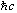197.326 960 1(78) MeV fm (= eV nm) 39 electron mass me 0.510 998 902(21) MeV/c2 = 9.109 381 88(72)´10–31 kg 40, 79 proton mass mp 938.271 998(38) MeV/c2 = 1.672 621 58(13)´10–27 kg 40, 79 = 1.007 276 466 88(13) u = 1836.152 667 5(39) me 0.13, 2.1 deuteron mass md 1875.612 762 (75) MeV/c2 40 unified atomic mass unit (u) (mass 12C atom)/12 = (1 g)/(NA mol) 931.494 013(37) MeV/c2 = 1.660 538 73(13)´10–27 kg 40, 79 permittivity of free space e0 = 1/(m0c2) 8.854 187 817¼´10–12 F m–1 exact permeability of free space m0 4p´10–7 N A–2 = 12.566 370 614¼´10–7 N A–2 exact fine-structure constant a = e2/4pe01/137.035 999 76(50) 3.7 classical electron radius re = e2/4pe0mec2 2.817 940 285(31)´10–15 m 11 Bohr radius (mnucleus = ¥) a0 = 4pe0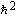/mee2 = rea–2 0.529 177 208 3(19)´10–10 m (10–8 cm) 3.7 Rydberg energy hcR¥ = mee4/2(4pe0)2=mec2a2/2 13.605 691 72(53) eV 39 Thomson cross section sT = 8p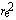/3 0.665 245 854(15) barn (10–28 m2) 22 Bohr magneton mB = e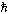/2me 5.788 381 749(43)´10–11 MeV T–1 7.3 nuclear magneton mN = e/2mp 3.152 451 238(24)´10–14 MeV T–1 7.6 electron cyclotron freq./field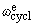/B = e/me 1.758 820 174(71)´1011 rad s–1 T–1 40 proton cyclotron freq./field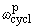/B = e/mp 9.578 834 08(38)´107 rad s–1 T–1 40

 Table 5-1. Physical constants(continued). Quantity Symbol, equation Value Uncert. (ppb) Avogadro constant NA 6.022 141 99(47)´1023 mol–1 79 Boltzman constant k 1.380 650 3(24)´10–23 J K–1 = 8.617 342(15)´10–5 eV K–1 1700 molar volume, ideal gas at STP NAk (273.15 K)/(101 325 Pa) 22.413 996(39)´10–3 m3 mol–1 1700 p = 3.141 592 653 589 793 238 e = 2.718 281 828 459 045 235 g = 0.577 215 664 901 532 861 The meter is the length of the path traveled by light in vacuum during a time interval of 1/299 792 458 of a second. 1 in.     =     2.54 cm 1 newton    =     105 dyne 1 eV/c2      =     1.782 662´10–33 g 1 coulomb      =       2.997 924 58´109 esu 1 Å     =     10–8 cm 1 joule       =     107 erg hc/(1 eV)       =     1.239 842 mm 1 tesla         =       104 gauss 1 fm     =     10–13 cm 1 cal       =     4.184 joule 1 eV/h       =     2.417 989´1014 Hz 1 atm         =       1.013 25´106 dyne/cm2 1 barn     =     10–24 cm2 1 eV       =     1.602 176 5´10–12 erg 1 eV/k      =     11 604.5 K 0°C         =       273.15 K